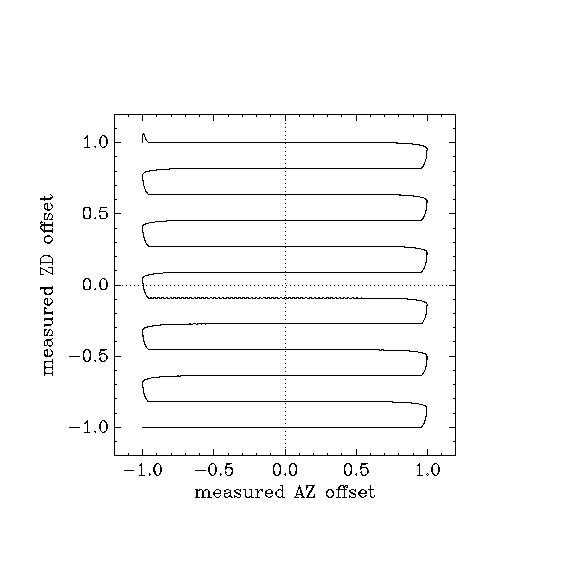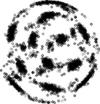<< WebHome

## What the problem was

• The problem was that scanning (2006) was not as regular as it was hoped to be:

## horizontal scanning in AZ-ZD (azimuth-zenith_distance) coordinates

### version of rt_main.c 2006-12-09 15:46

• rt_main.c 2006-12-09 15:46:### with scan_corners-0.1.patch

#### a few comments on the patch

The main change in the algorithm was to use the measured offsets for making decisions instead of the set (requested) offsets.

test_scan.c at present uses an arbitrary algorithm for modelling how the telescope actually gets to where it wants to get, and shakes the telescope around a bit to possibly detect any obvious numerical rounding problems.

SCAN_CORNER_TOLERANCE_DEG in rt_main.h decides how sharp or rounded the corners should be - the smaller it is, the more sharp they are.

• scan_patch_0.1_080612hor.png:parameter in rt_main.h: #define SCAN_CORNER_TOLERANCE_DEG 0.002 /* tolerance in degrees for scans */

parameters for scan_patch_0.1_080612hor.png read from simulator:
```2008.6.12  15:40:21.639771 epoch=1950
ST=37195.7 UT=56421.6 date=1.2062e+07 JD=2.45463e+06 dayOfYear=164
real: AZ=29.0388 ZD=19.2727 REC=143.624 DEC=35.559
set:  AZ=30.0362 ZD=20.2732 REC=142.796 DEC=34.7486
offsets: AZ=-1 ZD=-1 REC=0.8285 DEC=0.8097
VA64 VZ=17
flags: Hoffset=1 Eoffset=0 Sourcedemand=1 Pointdemand=0
rCA=3840 rCB=3840 rCX=3840 rCY=3840 rCZ=3840
commandDesc :scan 1000 11  [OK]
```

#### identical code, but increase tolerance to: SCAN_CORNER_TOLERANCE_DEG 0.05

• scan_patch_0.1_tol0p05_080612.png:```2008.6.12  19:5:36.541391 epoch=1950
ST=49544.3 UT=68736.5 date=1.2062e+07 JD=2.45463e+06 dayOfYear=164
real: AZ=0.7797 ZD=17.8834 REC=206.142 DEC=35.2133
set:  AZ=2.0841 ZD=18.391 REC=206.136 DEC=34.7132
offsets: AZ=-1 ZD=-1 REC=0.4007 DEC=0.9935
VA74 VZ=1
flags: Hoffset=1 Eoffset=0 Sourcedemand=1 Pointdemand=0
rCA=3840 rCB=3840 rCX=3840 rCY=3840 rCZ=3840
commandDesc :scan 1000 11  [OK]
```

-- BoudRoukema - 17 Jun 2008
Topic revision: r2 - 20 Jun 2008, BoudRoukemaEnglish FranĂ§ais Polski
Copyright © CC-BY-SA by the contributing authors. All material on this collaboration platform is copyrighted under CC-BY-SA by the contributing authors unless otherwise noted.
Ideas, requests, problems regarding Foswiki? Send feedback